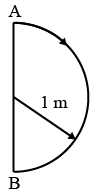# ISRO Scientist or Engineer Mechanical 2016

Instructions

For the following questions answer them individually

Question 21

# A thermometer works on the principle ofQuestion 22

# The working temperatures in evaporator and condensercoils of a refrigerator are $$-25^\circ C$$ and $$30^\circ C$$, respectively. The COP of the refrigerator is 0.85 of the maximum COP for a power input of 2 kW. The refrigeration effect produced will beQuestion 23

# A mercury thermometer was first placed in melting ice and the length of mercury column was observed to be 10 mm; when it was placed in steam, the length of the column was250 mm. Whenplaced in tap water, the length of the column was 58 mm. The temperature of the tap water isQuestion 24

# The amountof steam (at $$100^\circ C$$) required to raise the temperature of 200 g of water from $$60^\circ C$$ to $$100^\circ C$$ isQuestion 25

# A engine works on Carnot cycle between $$727^\circ C$$ and $$227^\circ C$$. The efficiency of the engine isQuestion 26

# A steam thermal powerplant works onQuestion 27

# A boat which has a speed of 5 km/h in still water crosses a river of width 1 km along the shortest path in 15 min. The velocity of the river in km/h isQuestion 28

# In 1s, a particle goes from point A to point B, moving in a semicircle (as shown in figure). The magnitude of the average velocity isQuestion 29

# A block is made to slide down an inclined plane ($$30^\circ$$ with horizontal) which is smooth. It starts sliding from rest and takes a time ‘t’ to reach the bottom of the plane. An identical bodyis freely dropped from the same point. The time the body takes to reach the bottom isQuestion 30

# What parameterwill remain constant in a throttling process?OR